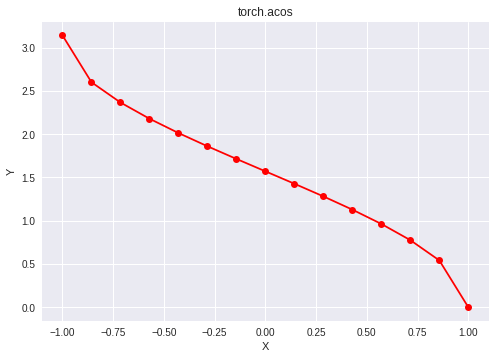# Python | PyTorch acos() method

• Last Updated : 07 Jan, 2022

PyTorch is an open-source machine learning library developed by Facebook. It is used for deep neural network and natural language processing purposes.

The function `torch.acos()` provides support for the inverse cosine function in PyTorch. It expects the input to be in the range [-1, 1] and gives the output in radian form. It returns nan if the input does not lie in the range [-1, 1]. The input type is tensor and if the input contains more than one element, element-wise inverse cosine is computed.

Syntax: torch.acos(x, out=None)

Parameters:
x: Input tensor
name (optional): Output tensor

Return type: A tensor with the same type as that of x.

Code #1:

## Python3

 `# Importing the PyTorch library ` `import` `torch ` ` `  `# A constant tensor of size 6 ` `a ``=` `torch.FloatTensor([``1.0``, ``-``0.5``, ``3.4``, ``0.2``, ``0.0``, ``-``2``]) ` `print``(a) ` ` `  `# Applying the inverse cos function and ` `# storing the result in 'b' ` `b ``=` `torch.acos(a) ` `print``(b) `

Output:

```tensor([ 1.0000, -0.5000,  3.4000,  0.2000,  0.0000, -2.0000])
tensor([0.0000, 2.0944,    nan, 1.3694, 1.5708,    nan])
```

Code #2: Visualization

## Python3

 `# Importing the PyTorch library ` `import` `torch ` ` `  `# Importing the NumPy library ` `import` `numpy as np ` ` `  `# Importing the matplotlib.pyplot function ` `import` `matplotlib.pyplot as plt ` ` `  `# A vector of size 15 with values from -1 to 1 ` `a ``=` `np.linspace(``-``1``, ``1``, ``15``) ` ` `  `# Applying the inverse cosine function and ` `# storing the result in 'b' ` `b ``=` `torch.acos(torch.FloatTensor(a)) ` ` `  `print``(b) ` ` `  `# Plotting ` `plt.plot(a, b.numpy(), color ``=` `'red'``, marker ``=` `"o"``)  ` `plt.title(``"torch.acos"``)  ` `plt.xlabel(``"X"``)  ` `plt.ylabel(``"Y"``)  ` ` `  `plt.show() `

Output:

```tensor([3.1416, 2.6005, 2.3664, 2.1790, 2.0137, 1.8605, 1.7141, 1.5708, 1.4274,
1.2810, 1.1279, 0.9626, 0.7752, 0.5411, 0.0000])
```My Personal Notes arrow_drop_up
Recommended Articles
Page :